## Characteristics of Rational Functions

### Learning Outcomes

• Use arrow notation to describe local and end behavior of rational functions.
• Identify horizontal and vertical asymptotes of rational functions from graphs.
• Graph a rational function given horizontal and vertical shifts.
• Write a rational function that describes mixing.

We have seen the graphs of the basic reciprocal function and the squared reciprocal function from our study of toolkit functions. Examine these graphs and notice some of their features.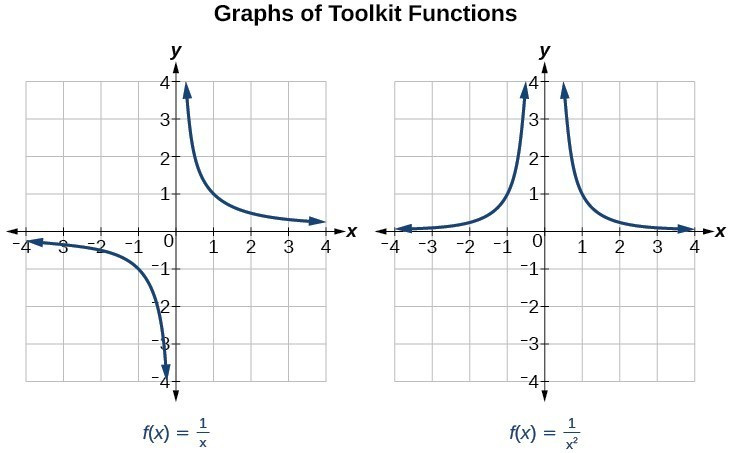Several things are apparent if we examine the graph of $f\left(x\right)=\dfrac{1}{x}$.

1. On the left branch of the graph, the curve approaches the $x$-axis $\left(y=0\right) \text{ as } x\to -\infty$.
2. As the graph approaches $x=0$ from the left, the curve drops, but as we approach zero from the right, the curve rises.
3. Finally, on the right branch of the graph, the curves approaches the $x$axis $\left(y=0\right) \text{ as } x\to \infty$.

To summarize, we use arrow notation to show that $x$ or $f\left(x\right)$ is approaching a particular value.

Arrow Notation
Symbol Meaning
$x\to {a}^{-}$ $x$ approaches $a$ from the left ($x<a$ but close to $a$)
$x\to {a}^{+}$ $x$ approaches $a$ from the right ($x<a$ but close to $a$)
$x\to \infty$ $x$ approaches infinity ($x$ increases without bound)
$x\to -\infty$ $x$ approaches negative infinity ($x$ decreases without bound)
$f\left(x\right)\to \infty$ the output approaches infinity (the output increases without bound)
$f\left(x\right)\to -\infty$ the output approaches negative infinity (the output decreases without bound)
$f\left(x\right)\to a$ the output approaches $a$

## Local Behavior of $f\left(x\right)=\frac{1}{x}$

Let’s begin by looking at the reciprocal function, $f\left(x\right)=\frac{1}{x}$. We cannot divide by zero, which means the function is undefined at $x=0$; so zero is not in the domain.

As the input values approach zero from the left side (becoming very small, negative values), the function values decrease without bound (in other words, they approach negative infinity). We can see this behavior in the table below.

 $x$ –0.1 –0.01 –0.001 –0.0001 $f\left(x\right)=\frac{1}{x}$ –10 –100 –1000 –10,000

We write in arrow notation:

$\text{as }x\to {0}^{-},f\left(x\right)\to -\infty$

As $x$ approaches $0$ from the left (negative) side, $f(x)$ will approach negative infinity.

As the input values approach zero from the right side (becoming very small, positive values), the function values increase without bound (approaching infinity). We can see this behavior in the table below.

 $x$ 0.1 0.01 0.001 0.0001 $f\left(x\right)=\frac{1}{x}$ 10 100 1000 10,000

We write in arrow notation:

$\text{As }x\to {0}^{+}, f\left(x\right)\to \infty$.

As $x$ approaches $0$ from the right (positive) side, $f(x)$ will approach infinity.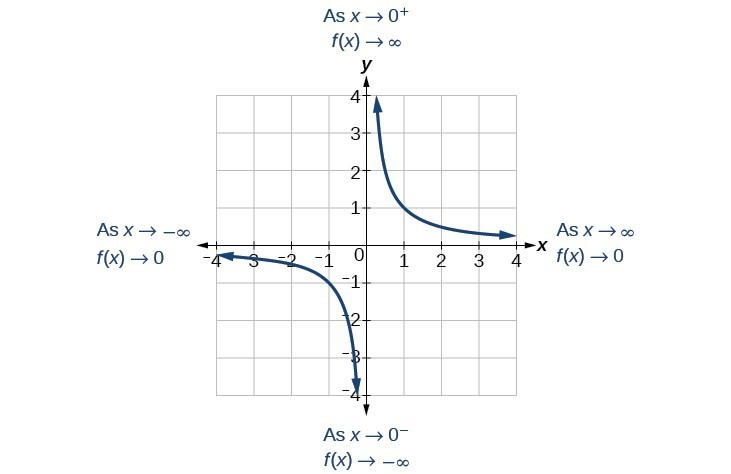This behavior creates a vertical asymptote, which is a vertical line that the graph approaches but never crosses. In this case, the graph is approaching the vertical line $x=0$ as the input becomes close to zero.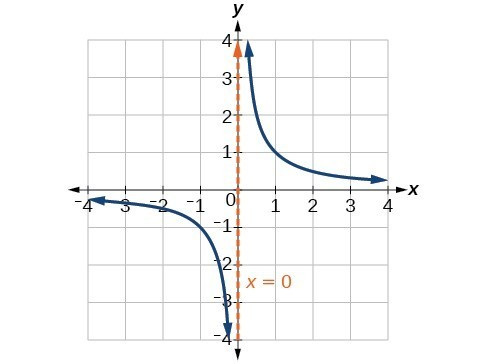### A General Note: Vertical Asymptote

A vertical asymptote of a graph is a vertical line $x=a$ where the graph tends toward positive or negative infinity as the inputs approach $x$. We write

$\text{As }x\to a,f\left(x\right)\to \infty , \text{or as }x\to a,f\left(x\right)\to -\infty$.

## End Behavior of $f\left(x\right)=\frac{1}{x}$

As the values of $x$ approach infinity, the function values approach 0. As the values of $x$ approach negative infinity, the function values approach 0. Symbolically, using arrow notation

$\text{As }x\to \infty ,f\left(x\right)\to 0,\text{and as }x\to -\infty ,f\left(x\right)\to 0$.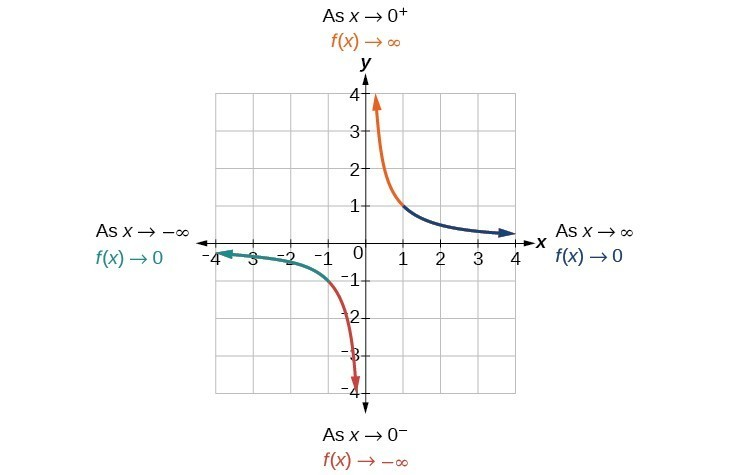Based on this overall behavior and the graph, we can see that the function approaches 0 but never actually reaches 0; it seems to level off as the inputs become large. This behavior creates a horizontal asymptote, a horizontal line that the graph approaches as the input increases or decreases without bound. In this case, the graph is approaching the horizontal line $y=0$.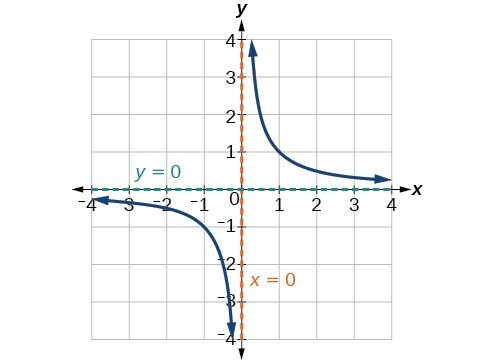### A General Note: Horizontal Asymptote

A horizontal asymptote of a graph is a horizontal line $y=b$ where the graph approaches the line as the inputs increase or decrease without bound. We write

$\text{As }x\to \infty \text{ or }x\to -\infty ,\text{ }f\left(x\right)\to b$.

### Example: Using Arrow Notation

Use arrow notation to describe the end behavior and local behavior of the function below.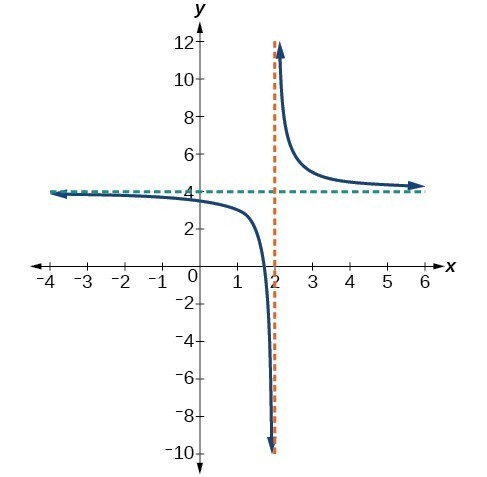### Try It

Use arrow notation to describe the end behavior and local behavior for the reciprocal squared function.

### Example: Using Transformations to Graph a Rational Function

Sketch a graph of the reciprocal function shifted two units to the left and up three units. Identify the horizontal and vertical asymptotes of the graph, if any.

### Try It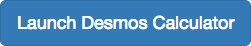Sketch the graph, and find the horizontal and vertical asymptotes of the reciprocal squared function that has been shifted right 3 units and down 4 units.

## A Mixing Problem

In the previous example, we shifted a toolkit function in a way that resulted in the function $f\left(x\right)=\dfrac{3x+7}{x+2}$. This is an example of a rational function. A rational function is a function that can be written as the quotient of two polynomial functions. Many real-world problems require us to find the ratio of two polynomial functions. Problems involving rates and concentrations often involve rational functions.

### A General Note: Rational Function

A rational function is a function that can be written as the quotient of two polynomial functions $P\left(x\right) \text{and} Q\left(x\right)$.

$f\left(x\right)=\dfrac{P\left(x\right)}{Q\left(x\right)}=\dfrac{{a}_{p}{x}^{p}+{a}_{p - 1}{x}^{p - 1}+…+{a}_{1}x+{a}_{0}}{{b}_{q}{x}^{q}+{b}_{q - 1}{x}^{q - 1}+…+{b}_{1}x+{b}_{0}},Q\left(x\right)\ne 0$

### Example: Solving an Applied Problem Involving a Rational Function

A large mixing tank currently contains 100 gallons of water into which 5 pounds of sugar have been mixed. A tap will open pouring 10 gallons per minute of water into the tank at the same time sugar is poured into the tank at a rate of 1 pound per minute. Find the concentration (pounds per gallon) of sugar in the tank after 12 minutes. Is that a greater concentration than at the beginning?

### Try It

There are 1,200 freshmen and 1,500 sophomores at a prep rally at noon. After 12 p.m., 20 freshmen arrive at the rally every five minutes while 15 sophomores leave the rally. Find the ratio of freshmen to sophomores at 1 p.m.

## Contribute!

Did you have an idea for improving this content? We’d love your input.